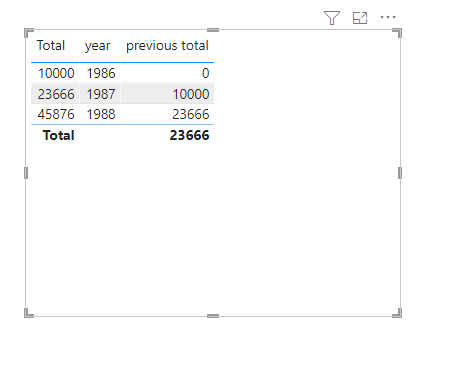cancel
Showing results for
Did you mean:Frequent Visitor

## Show % change for total yoy

I have a table which shows only totals per year, and i needto show the percentage change/growth on the same graph

What is the measure for that and which is the approriate graph type? e.g. year vs sales for each year

e.g. Table "total"

 year Total 1986 10000 1987 23666 1988 45876

Also, i have a table that lists only entries for employees (acheivments) (Only i can ghow can i calculate day/month/year % change for this, with card showing the values in red - / or green + (depends if the value is less or more that the previos selected day/month/year

 Date Achievments 12-01-2021 New project 13-01-2021 Employee of the month 14-01-2021 Cost reduction initiative

The idea is to monitor the changes/% for staff

all the queries here answers the changes for sum, but i din't find any solution for the total count

1 ACCEPTED SOLUTIONCommunity Support

You can calculate the total for the previous year as

``previous total = CALCULATE(SUM('Table'[Total]),FILTER(ALLSELECTED('Table'),[year]=MAX('Table'[year])-1))+0``The percent change is then calculated as follows.

``percentage = DIVIDE(SUM('Table'[Total])-[previous total],[previous total])+0``You can try the stacked column chart to dispaly.Best Regards,

Stephen Tao

If this post helps, then please consider Accept it as the solution to help the other members find it more quickly.Community Support

You can calculate the total for the previous year as

``previous total = CALCULATE(SUM('Table'[Total]),FILTER(ALLSELECTED('Table'),[year]=MAX('Table'[year])-1))+0``The percent change is then calculated as follows.

``percentage = DIVIDE(SUM('Table'[Total])-[previous total],[previous total])+0``You can try the stacked column chart to dispaly.Best Regards,

Stephen Tao

If this post helps, then please consider Accept it as the solution to help the other members find it more quickly.